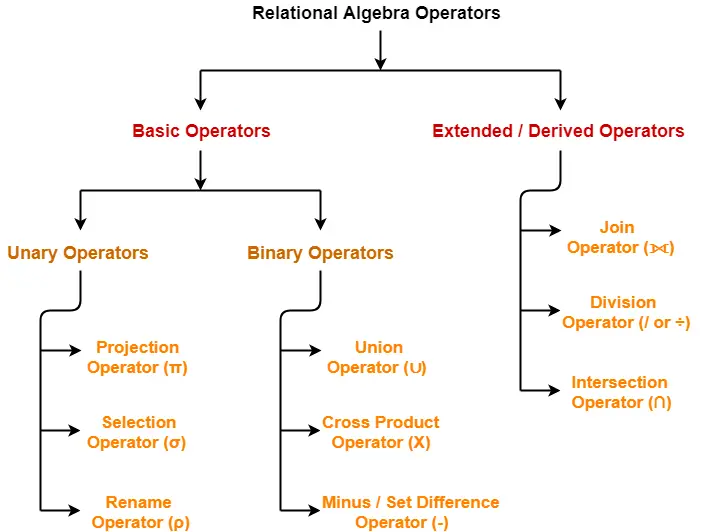## Relational Algebra Operators-

Before you go through this article, make sure that you have gone through the previous article on Introduction to Relational Algebra.

The operators in relational algebra are classified as-## Selection Operator-

• Selection Operator (σ) is a unary operator in relational algebra that performs a selection operation.
• It selects those rows or tuples from the relation that satisfies the selection condition.

 σ(R)

## Examples-

• Select tuples from a relation “Books” where subject is “database”

σsubject = “database” (Books)

• Select tuples from a relation “Books” where subject is “database” and price is “450”

σsubject = “database” ∧ price = “450” (Books)

• Select tuples from a relation “Books” where subject is “database” and price is “450” or have a publication year after 2010

σsubject = “database” ∧ price = “450” ∨ year >”2010″ (Books)

## Point-01:

• We may use logical operators like ∧ , ∨ , ! and relational operators like  = , ≠ , > , < , <= , >= with the selection condition.

## Point-02:

• Selection operator only selects the required tuples according to the selection condition.
• It does not display the selected tuples.
• To display the selected tuples, projection operator is used.

## Point-03:

• Selection operator always selects the entire tuple. It can not select a section or part of a tuple.

## Point-04:

• Selection operator is commutative in nature i.e.

σ A ∧ B (R) = σ B ∧ A (R)

OR

σ (σ A(R)) = σ (σ B(R))

## Point-05:

• Degree of the relation from a selection operation is same as degree of the input relation.

## Point-06:

• The number of rows returned by a selection operation is obviously less than or equal to the number of rows in the original table.

Thus,

• Minimum Cardinality = 0
• Maximum Cardinality = |R|

Next Article- Projection Operation in Relational Algebra

Get more notes and other study material of Database Management System (DBMS).

Watch video lectures by visiting our YouTube channel LearnVidFun.